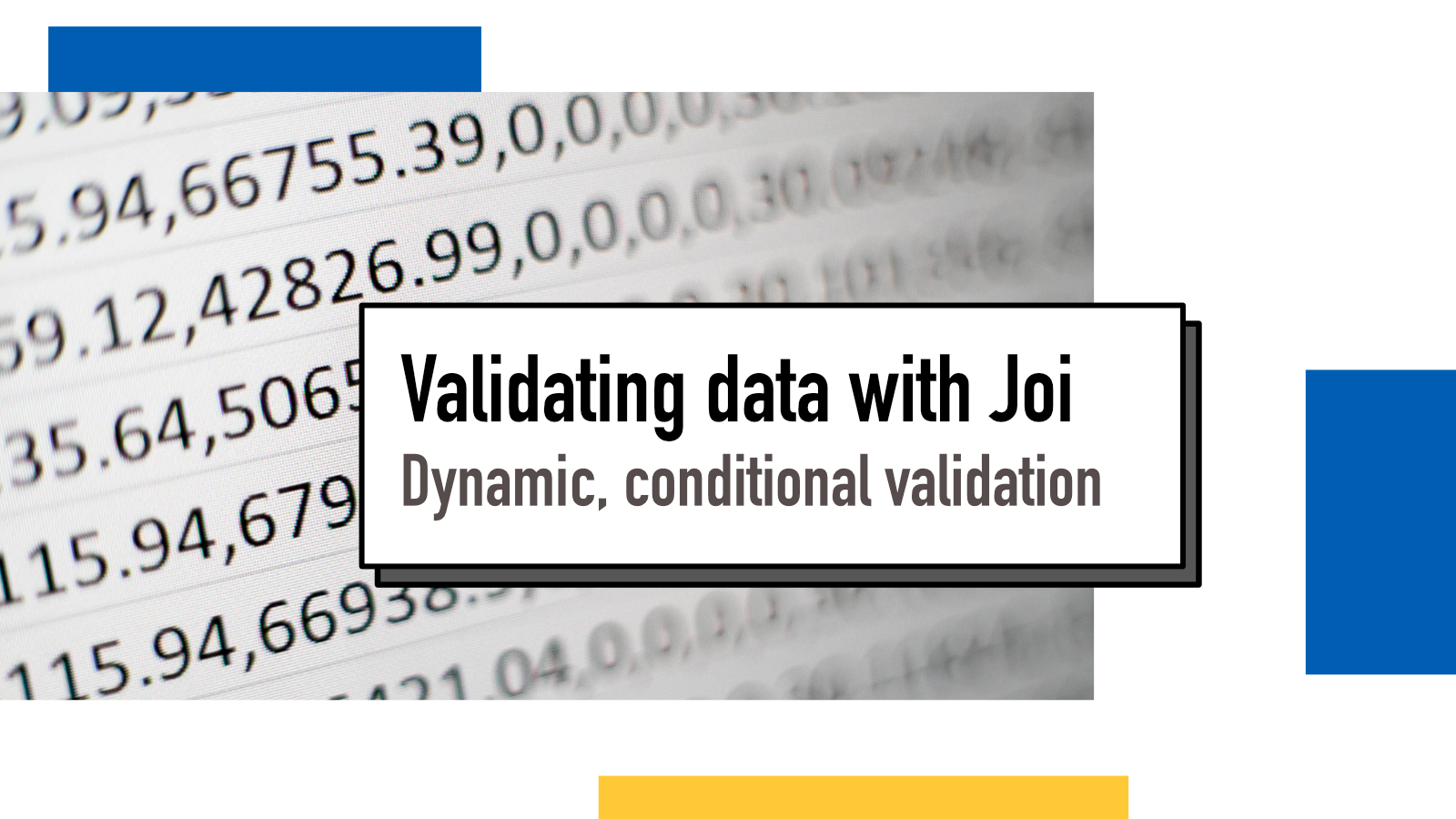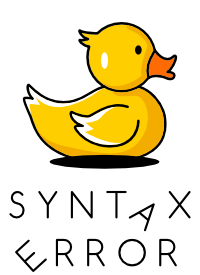Juha-Matti Santala

# Validating dynamic data conditionally with JoiI ran into an interesting case of data validation with Joi last week and since I couldn't find a clear answer on the Internet, I figured I'd write down my exploration and a working example.

## The problem

A friend asked help with a problem of creating a validation schema. They had an object with unknown/dynamic keys and string values (see example below). For all these values, they wanted to validate according to these rules:

1. If the value is a string that converts into a NaN when trying to convert to number, accept any string
2. If the value is a string that converts into a valid number, accept only numbers that are positive or zero (>= 0).

Doing this type of conditional validation can be a bit tricky with Joi. There are many ways to do different conditionals but some only work in certain cases.

Let's take a look at an example data:

``````{
"name": "Sherlock Holmes",
"successRatio": "0.9",
"age": "34"
}``````

Here, we want to pass name and address to always pass but want `successRatio` and `age` to be validated and to be positive or zero.

## Schema

After tinkering a bit in the Joi.dev Sandbox, I eventually ended up with this:

``````Joi
.object()
.pattern(/./,
Joi.alternatives().conditional(
Joi.number(),
{
then: Joi.number().min(0),
otherwise: Joi.string()
}
)
)``````

For every value, it runs a `conditional`. The first argument of a conditional is a test: in this case we check if the value is numeric (or converts to a numeric value). The second argument is an options object with two values: `then` and `otherwise` which are basically if/else blocks. For values that are numeric, we check that they are at least 0 and for values that are not numeric, we just check that they are strings.

With this schema and the above example data, it should pass. If you wanna see it fail, change `successRate` to a negative value.